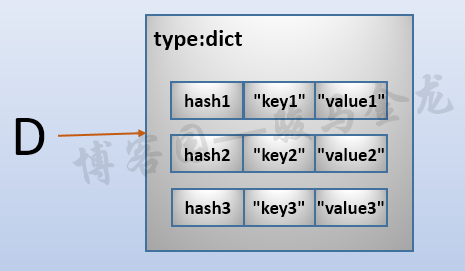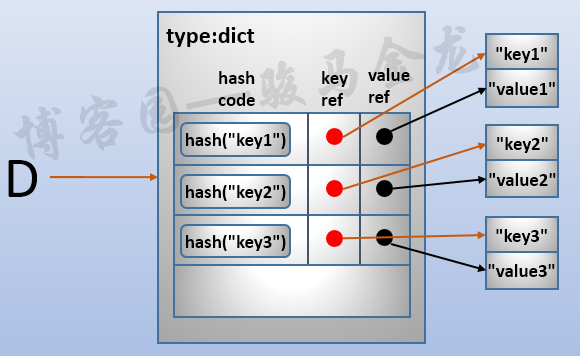# python字典类型

## 字典类型简介

D = {"key1": "value1",
"key2": "value2",
"key3": "value3"}


dict对象中存储的元素没有位置顺序，所以dict不是序列，不能通过索引的方式取元素。dict是按照key进行存储的，所以需要通过key作为定位元素的依据，比如取元素或修改key对应的value。比如：

D['key1']     # 得到value1
D['key2']     # 得到value2
D['key3']     # 得到value3


## 字典的结构

dict是一个hashtable数据结构，除了数据类型的声明头部分，还主要存储了3部分数据：一个hash值，两个指针。下面详细解释dict的结构。

D = {"key1": "value1",
"key2": "value2",
"key3": "value3"}typedef struct {
/* Cached hash code of me_key. */
Py_hash_t me_hash;
PyObject *me_key;
PyObject *me_value;
} PyDictKeyEntry;dict是可变的，可以删除元素、增加元素、修改元素的value。这些操作的过程与上面的过程类似，都是先hash，并根据hash值来存储或检索元素。

# 字符串作为key
>>> D = {"aa":"aa","bb":"bb"}
>>> D
{'aa': 'aa', 'bb': 'bb'}

# 数值作为key
>>> D = {1:"aa","bb":"bb"}
>>> D
'aa'

# 元组作为key
>>> D = {(1,2):"aa","bb":"bb"}
>>> D
{(1, 2): 'aa', 'bb': 'bb'}

# 列表作为key，报错
>>> D = {[1,2]:"aa","bb":"bb"}
Traceback (most recent call last):
File "<stdin>", line 1, in <module>
TypeError: unhashable type: 'list'


### 字典元素的顺序改变

# python 3.5.2
>>> d = {'one': 1, 'two': 2, 'three': 3, 'four': 4}
>>> d
{'four': 4, 'two': 2, 'three': 3, 'one': 1}


Changed in version 3.7: Dictionary order is guaranteed to be insertion order. This behavior was an implementation detail of CPython from 3.6.

# python 3.7.1
>>> d = {'one': 1, 'two': 2, 'three': 3, 'four': 4}
>>> d
{'one': 1, 'two': 2, 'three': 3, 'four': 4}


### 字典和列表的比较

python中list是元素有序存储的序列代表，dict是元素无序存储的代表。它们都可变，是python中最灵活的两种数据类型。

1. dict的元素检索、增删改速度快，不会随着元素增多、减少而改变。但缺点是内存占用大
2. list的元素检索、增删改速度随着元素增多会越来越慢(当然实际影响并没有多大)，但是内存占用小

## 构造字典

1. 使用大括号包围
2. 使用dict()构造方法，dict()构造有3种方式：
• dict(key=value)
• dict(DICT)
• dict(iterable)，其中iterable的每个元素必须是两元素的数据对象，例如("one",1)["two",2]
• 后两种都可以结合第一种方式
3. 使用dict对象的fromkey()方法
4. 使用dict对象的copy()方法
5. 字典解析的方式。这个在后文再解释
>>> D = {}     # 空字典
>>> type(D)
<class 'dict'>

>>> D = {"key1": "value1",
"key2": "value2",
"key3": "value3"}
>>> D
{'key1': 'value1', 'key2': 'value2', 'key3': 'value3'}

>>> a = dict(one=1, two=2, three=3)
>>> b = {'one': 1, 'two': 2, 'three': 3}
>>> c = dict(zip(['one', 'two', 'three'], [1, 2, 3]))
>>> d = dict([('two', 2), ('one', 1), ('three', 3)])
>>> e = dict({'three': 3, 'one': 1, 'two': 2})
>>> f = dict([('two', 2), ('one', 1), ('three', 3)], four=4, five=5)


fromkey(seq,value)是dict的类方法，所以可直接通过dict类名来调用(当然，使用已存在的对象来调用也没有问题)。它构造的字典的key来自于给定的序列，值来自于指定的第二个参数，如果没有第二个参数，则所有key的值默认为None。所以，第二个参数是构造新dict时的默认值。

>>> dict.fromkeys(range(5))
{0: None, 1: None, 2: None, 3: None, 4: None}

>>> dict.fromkeys(range(5), "aa")
{0: 'aa', 1: 'aa', 2: 'aa', 3: 'aa', 4: 'aa'}


>>> d = dict(one=1, two=2, three=3, four=4, five=5)

>>> dict.fromkeys(d)
{'one': None, 'two': None, 'three': None, 'four': None, 'five': None}

>>> dict.fromkeys(d, "aa")
{'one': 'aa', 'two': 'aa', 'three': 'aa', 'four': 'aa', 'five': 'aa'}


>>> dict.fromkeys("abcd","aa")
{'a': 'aa', 'b': 'aa', 'c': 'aa', 'd': 'aa'}

>>> L = ["a", "b", "c", "d"]
>>> dict.fromkeys(L)
{'a': None, 'b': None, 'c': None, 'd': None}

>>> T = ("a", "b", "c", "d")
>>> dict.fromkeys(L)
{'a': None, 'b': None, 'c': None, 'd': None}


dict的copy()方法会根据已有字典完全拷贝成一个新的字典副本。但需要注意的是，拷贝过程是浅拷贝。

>>> d = {'one': 1, 'two': 2, 'three': 3, 'four': 4}
>>> dd = d.copy()
>>> dd
{'three': 3, 'one': 1, 'two': 2, 'four': 4}

>>> id(d["one"]), id(dd["one"])
(10919424, 10919424)


## 操作字典

### dict的增删改查

>>> d
{'one': 1, 'two': 2, 'three': 3}

>>> d["one"]
1

>>> d["four"] = 4
>>> d
{'one': 1, 'two': 2, 'three': 3, 'four': 4}

>>> d["ten"]
Traceback (most recent call last):
File "<stdin>", line 1, in <module>
KeyError: 'ten'


>>> d = {'one': 1, 'two': 2, 'three': 3, 'four': 4}

>>> class mydict(dict):
...     def __missing__(self, key):
...         return None
...

>>> dd = mydict(d)
>>> dd
{'one': 1, 'two': 2, 'three': 3, 'four': 4}
>>> dd["ten"]
>>> print(dd["ten"])
None


get(key,default)方法检索dict中的元素，如果元素存在，则返回对应的value，否则返回指定的default值，如果没有指定default，且检索的key又不存在，则返回None。这正好是上面自定义dict子类的功能。

>>> d = {'one': 1, 'two': 2, 'three': 3, 'four': 4}

>>> d.get("two")
2

>>> d.get("six","not exists")
'not exists'
>>> print(d.get("six"))
None


len()函数可以用来查看字典有多少个元素：

>>> d
{'three': 3, 'four': 4, 'two': 2, 'one': 1}
>>> len(d)
4


setdefault(key,default)方法检索并设置一个key/value，如果key已存在，则直接返回对应的value，如果key不存在，则新插入这个key并指定其value为default并返回这个default，如果没有指定default，key又不存在，则默认为None。

>>> d.setdefault("one")
1

>>> d.setdefault("five")
{'one': 1, 'two': 2, 'three': 3, 'four': 4, 'five': None}

>>> d.setdefault("six",6)
6
>>> d
{'one': 1, 'two': 2, 'three': 3, 'four': 4, 'five': None, 'six': 6}


update(key/value)方法根据给定的key/value对更新已有的键，如果键不存在则新插入。key/value的表达方式有多种，只要能表达出key/value的配对行为就可以。比如已有的dict作为参数，key=value的方式，2元素的迭代容器对象。

>>> d
{'one': 1, 'two': 2, 'three': 3, 'four': 4}

>>> d.update(five=5, six=6)   # key=value的方式
>>> d
{'one': 1, 'two': 2, 'three': 3, 'four': 4, 'five': 5, 'six': 6}

>>> d.update({"one":11, "two":22})  # dict作为参数
>>> d
{'one': 11, 'two': 22, 'three': 3, 'four': 4, 'five': 5, 'six': 6}

>>> d.update([("five",55),("six",66)])  # 列表中2元素的元组
>>> d
{'one': 11, 'two': 22, 'three': 3, 'four': 4, 'five': 55, 'six': 66}

>>> d.update((("five",55),("six",66)))  # 这些都可以
>>> d.update((["five",55],["six",66]))
>>> d.update(zip(["five","six"],[55,66]))


del D[KEY]可以用来根据key删除字典D中给定的元素，如果元素不存在则报错。

>>> d = {'one': 1, 'two': 2, 'three': 3, 'four': 4}

>>> del d["four"]
>>> d
{'three': 3, 'two': 2, 'one': 1}

>>> del d["five"]
Traceback (most recent call last):
File "<stdin>", line 1, in <module>
KeyError: 'five'


clear()方法用来删除字典中所有元素。

>>> d = {'three': 3, 'four': 4, 'two': 2, 'one': 1}
>>> d.clear()
>>> d
{}


pop(key,default)用来移除给定的元素并返回移除的元素。但如果元素不存在，则返回default，如果不存在且没有给定default，则报错。

>>> d = {'three': 3, 'four': 4, 'two': 2, 'one': 1}

>>> d.pop("one")
1
>>> d.pop("five","hello world")
'hello world'
>>> d.pop("five")
Traceback (most recent call last):
File "<stdin>", line 1, in <module>
KeyError: 'five'


popitem()用于移除并返回一个(key,value)元组对，每调用一次移除一个元素，没元素可移除后将报错。在python 3.7中保证以LIFO的顺序移除，在此之前不保证移除顺序。

>>> d
{'three': 3, 'four': 4, 'two': 2, 'one': 1}

>>> d.popitem()
('three', 3)
>>> d.popitem()
('four', 4)
>>> d.popitem()
('two', 2)
>>> d.popitem()
('one', 1)
>>> d.popitem()
Traceback (most recent call last):
File "<stdin>", line 1, in <module>
KeyError: 'popitem(): dictionary is empty'


### 测试

>>> "one" in d
True
>>> "one3" in d
False
>>> "one3" not in d
True


### 迭代和dict视图

• keys()返回字典中所有的key组成的视图对象；
• values()返回字典中所有value组成的视图对象；
• items()返回字典中所有(key,value)元组对组成的视图对象；
• iter(d)函数返回字典中所有key组成的可迭代对象。等价于iter(d.keys())

>>> d
{'three': 3, 'four': 4, 'two': 2, 'one': 1}

>>> d.keys()
dict_keys(['three', 'four', 'two', 'one'])
>>> list(d.keys())
['three', 'four', 'two', 'one']

>>> d.values()
dict_values([3, 4, 2, 1])

>>> d.items()
dict_items([('three', 3), ('four', 4), ('two', 2), ('one', 1)])


iter(d)返回的是由key组成的可迭代对象。

>>> iter(d)
<dict_keyiterator object at 0x7f0ab9c9c4f8>

>>> for i in iter(d):print(i)
...
three
four
two
one


>>> for i in d.keys():
...     print(i)
...
three
four
two
one

>>> for i in d.values():
...     print(i)
...
3
4
2
1

>>> for (key,value) in d.items():
...     print(key,"-->",value)
...
three --> 3
four --> 4
two --> 2
one --> 1


## dict视图对象

keys()、values()、items()返回字典视图对象。视图对象中的数据会随着原字典的改变而改变。如果知道关系型数据库里的视图，这很容易理解。

>>> d
{'one': 1, 'two': 2, 'three': 3, 'four': 4}
>>> d.keys()
dict_keys(['one', 'two', 'three', 'four'])

>>> list(d.keys())
['one', 'two', 'three', 'four']


>>> list(d.keys())
['one', 'two', 'three', 'four']


KEY in d.keys()

for key in d.keys(): ...
for value in d.values(): ...
for (key, value) in d.items(): ...


• len(obj_view)：返回视图对象的长度
• iter(obj_view)：返回视图对象对应的可迭代对象
>>> len(d.keys())
4

>>> iter(d.keys())
<dict_keyiterator object at 0x000001F0A7D9A9F8>


>>> d = {'one': 1, 'two': 2, 'three': 3, 'four': 4}

>>> ks = d.keys()

>>> del d["one"]
>>> k
dict_keys(['two', 'three', 'four'])


## 字典迭代和解析

for key in d:
for key in d.keys()


>>> d = {k:v for (k,v) in zip(["one","two","three"],[1,2,3])}
>>> d
{'one': 1, 'two': 2, 'three': 3}

>>> d = {x : x ** 2 for x in [1,2,3,4]}
>>> d
{1: 1, 2: 4, 3: 9, 4: 16}

>>> d = {x : None for x in "abcde"}
>>> d
{'a': None, 'b': None, 'c': None, 'd': None, 'e': None}

posted @ 2018-12-21 22:58  骏马金龙  阅读(1740)  评论(1编辑  收藏  举报